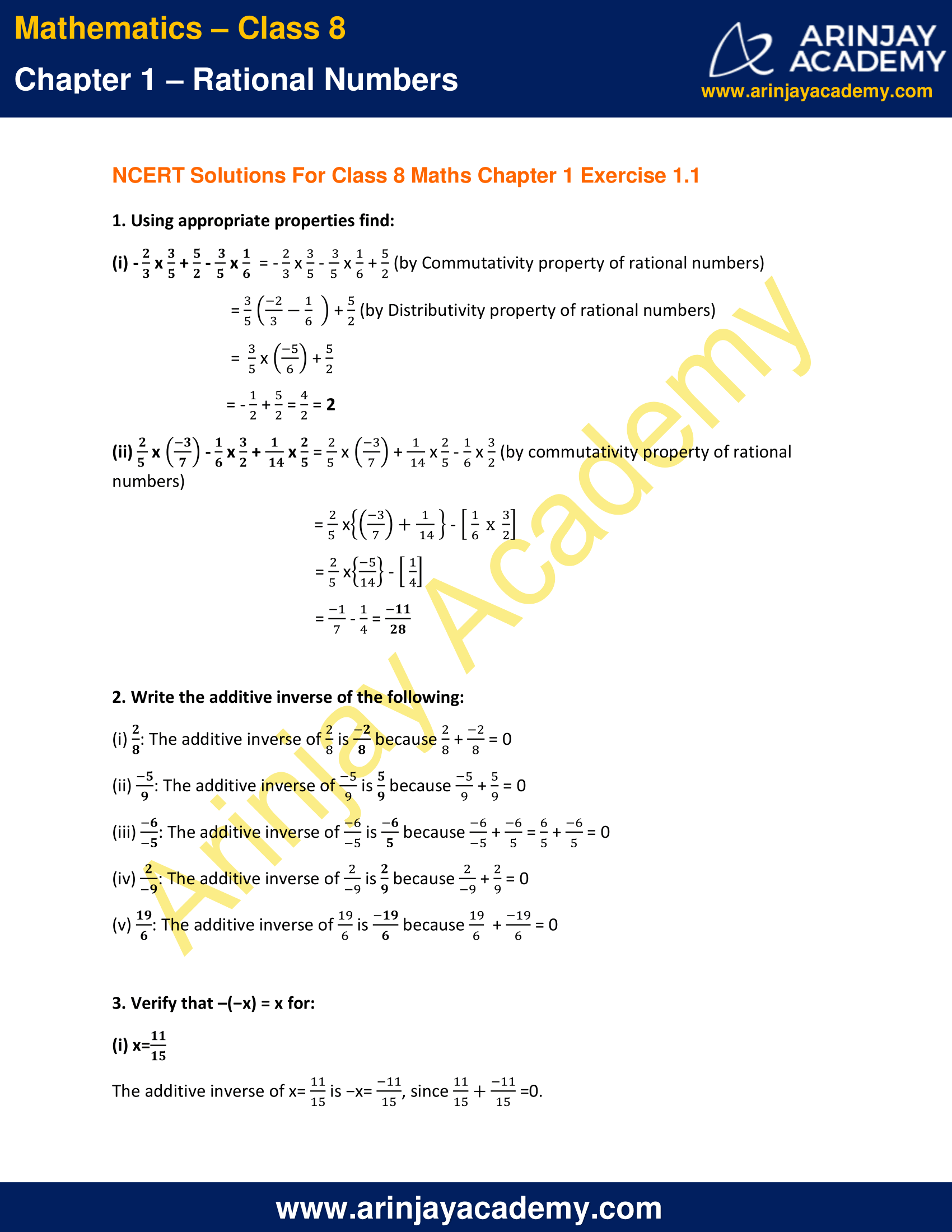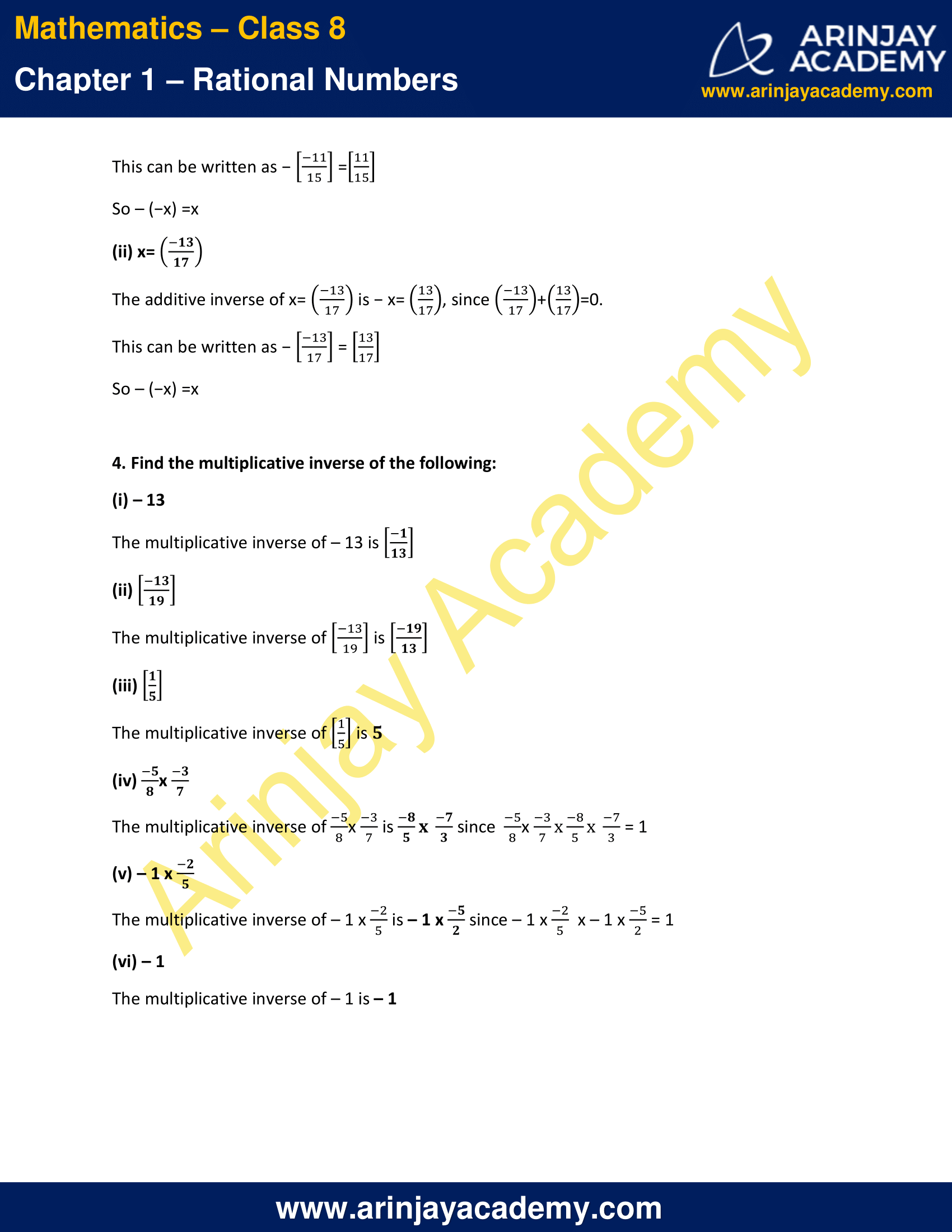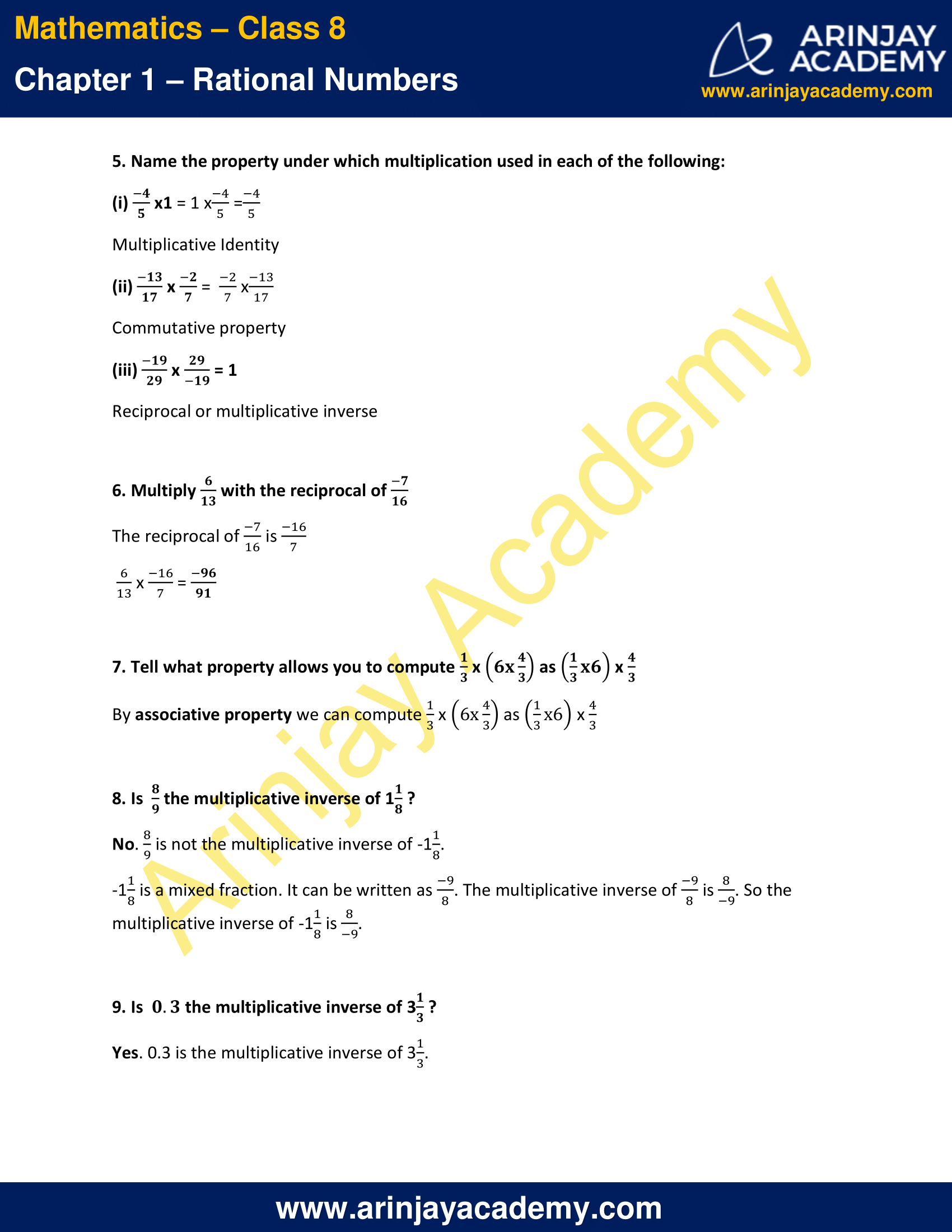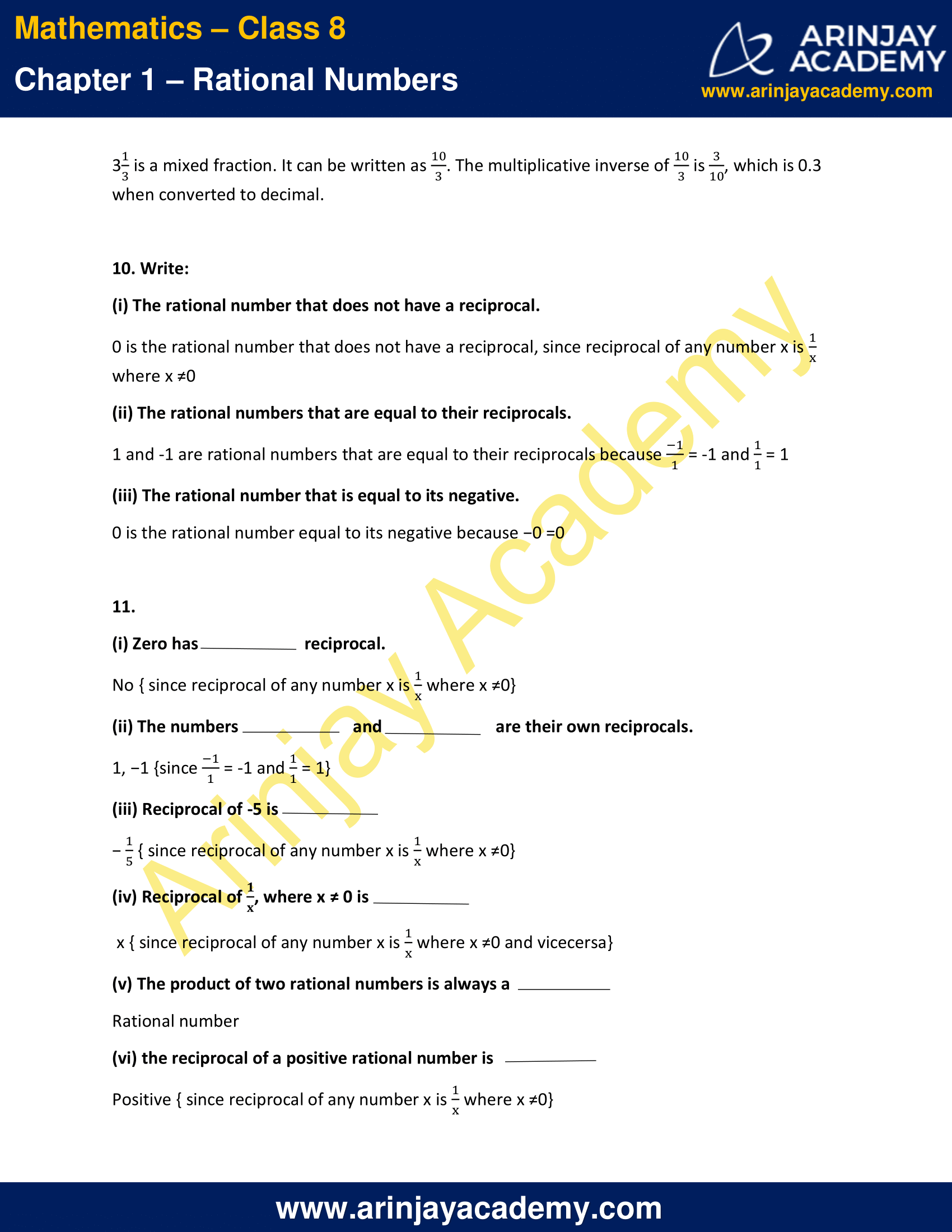# NCERT Solutions for Class 8 Maths Chapter 1 Exercise 1.1 – Rational Numbers

﻿NCERT Solutions for Class 8 Maths Chapter 1 Exercise 1.1 , has been designed by the NCERT to test the knowledge of the student on the following topics :

1.1 Introduction

1.2 Properties of Rational Numbers

• 1.2.1 Closure
Closure Property of Whole numbers
Closure Property of Integers
Closure Property of Rational Numbers
(b) Subtraction
(c) Multiplication
(d) Division
• 1.2.2 Commutativity
Commutative Property of Whole Numbers
Commutative Property of Integers
Commutative Property of Rational Numbers
(b) Subtraction
(c) Multiplication
(d) Division
• 1.2.3 Associativity
Associative Property of Whole Numbers
Associative Property of Integers
Associative Property of Rational Numbers
(b) Subtraction
(c) Multiplication
(d) Division
• 1.2.4 The role of zero (0)
• 1.2.5 The role of 1
• 1.2.6 Negative of a number
• 1.2.7 Reciprocal
• 1.2.8 Distributivity of multiplication over addition for rational numbers

### NCERT Solutions for Class 8 Maths Chapter 1 Exercise 1.1 – Rational NumbersThe next Exercise for NCERT Solutions for Class 8 Maths Chapter 1 Exercise 1.2 – Rational Numbers can be accessed by clicking here.